Functions and CALL Routines

# GARKHPTPRC Function

Calculates put prices for European options on stocks, based on the Garman-Kohlhagen model.
 Category: Financial

## Syntax

 GARKHPTPRC(E, t, S, Rd, Rf, sigma)

### Arguments

E

is a non-missing, positive value that specifies the exercise price.

 Requirement: Specify E and S in the same units.
t

is a non-missing value that specifies the time to maturity.

S

is a non-missing, positive value that specifies the spot currency price.

 Requirement: Specify S and E in the same units.
Rd

is a non-missing, positive fraction that specifies the risk-free domestic interest rate for period t.

 Requirement: Specify a value for Rd for the same time period as the unit of t.
Rf

is a non-missing, positive fraction that specifies the risk-free foreign interest rate for period t.

 Requirement: Specify a value for Rt for the same time period as the unit of t.
sigma

is a non-missing, positive fraction that specifies the volatility of the currency rate.

 Requirement: Specify a value for sigma for the same time period as the unit of t.

The GARKHPTPRC function calculates the put prices for European options on stocks, based on the Garman-Kohlhagen model. The function is based on the following relationship:
where

 S specifies the spot currency price. E specifies the exercise price of the option. t specifies the time to expiration. Rd specifies the risk-free domestic interest rate for period t. Rf specifies the risk-free foreign interest rate for period t.
where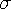specifies the volatility of the underlying asset.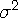specifies the variance of the rate of return.

For the special case of t=0, the following equation is true:
For information about the basics of pricing, see Using Pricing Functions.

The GARKHPTPRC function calculates the put prices for European options on stocks, based on the Garman-Kohlhagen model. The GARKHCLPRC function calculates the call prices for European options on stocks, based on the Garman-Kohlhagen model. These functions return a scalar value.

SAS Statements Results

`----+----1----+----2--`
```a=garkhptprc(1000, .5. 950, 4, 4, 2):
put a;```
`72.102451281`
```b=garkhptprc(850, 1.2. 125, 5, 3, 1):
put b;```
`0.5917507981`
```c=garkhptprc(7500, .9. 950, 3, 2, 2):
put c;```
`416.33604902`
```d=garkhptprc(5000, -.5, 237, 3, 3, 2):
put d;```
`           0`

 Function:Previous Page | Next Page | Top of Page